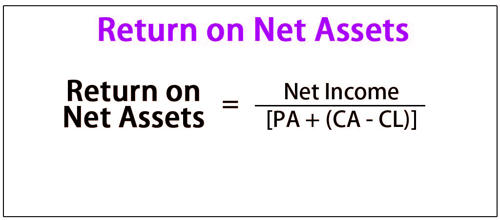The return on net assets (RONA) is a measure of the financial performance of a company that takes the use of assets into account. It is calculated by dividing a company’s net income in a given period by the total value of both its fixed assets and its working capital. It is a variation of the traditional return on assets ratio that uses fixed assets and net working capital in its calculation as opposed to total assets. It helps the investors to determine the percentage net income the company is generating from the assets. This ratio tells how effectively and efficiently the company is using its assets to generate earnings.

RONA measures how well a company’s fixed assets and net working capital perform in terms of generating net income. Higher RONA means that the company is using its assets and working capital efficiently and effectively. RONA is used by investors to determine how well management is utilizing assets. It is also used to assess how well a company is performing compared to others in its industry. The RONA ratio is used to determine the efficiency and effectiveness of a company’s use of its assets. This ratio should usually be used in capital-intensive industries where major purchases are for fixed assets.Basic formulae

Return on net assets = net income / (Fixed assets + working capital)

where Working capital = (current assets minus current liabilities)

In the manufacturing sector, this is also calculated as:

Return on net assets = (plant revenue – costs) / net assets

For example, suppose that Company XYZ owns, in a given period, \$500k in fixed assets accompanied by \$300k in working capital. In the same period, XYZ generates \$200k in net income. XYZ’s RONA would be calculated in the following way:

RONA = \$200,000 net income / (\$500,000 A Fixed + \$300,000 C Working)

= \$200,000 net income / \$800,000 A Fixed and C Working = 0.25 or 25%

In this instance, XYZ generated a 25% return on its working capital combined with its fixed assets.

The RONA ratio shows how well a company and its management are deploying assets in economically valuable ways, and a high ratio result indicates that management is squeezing more earnings out of each dollar invested in assets. Hence, it measures the efficiency of a company in generating returns on the assets it owns. Return on net assets is commonly used for capital-intensive companies and is an important ratio looked at by investors and analysts to determine how effective and efficient a company is at generating a profitable return on its net assets.